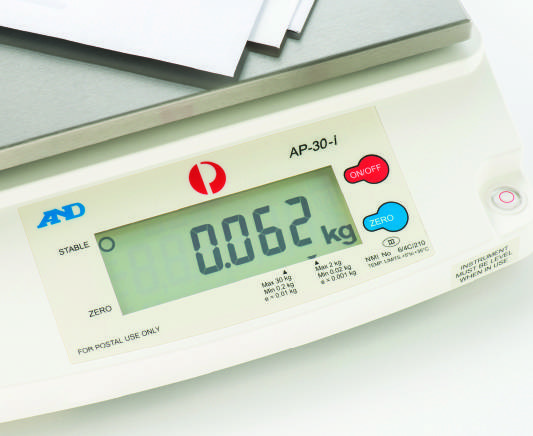# Work done in raising a weight

TL;DR Summary
Does the formula Mgh contain the acceleration due to gravity twice?
Hello

The formula Mgh is commonly accepted as the work done by raising a mass by a distance h, where M is defined as the mass of the object raised.

However, is this really the mass, or the weight, simply obtained by weighing the object? If it's the weight, then doesn't the equation effectively contain the acceleration due to gravity twice?

best regards ... Stef

Staff Emeritus
2022 Award
It's the mass of the object, not the weight.

•russ_watters
Many thanks.

So to be clear, I would have to weight the object and then divide this by 9.8 to get the mass?

Staff Emeritus
2022 Award
That's right.

•russ_watters
Gold Member
Most balances are calibrated in kg, so they are indicating mass. A true weight meter would give a calibration in Newtons. In such a case it is correct to divide by 9.8, but otherwise not.

•russ_watters
Ah OK, that's interesting, and confusing.

The scales I'm using are for weighing items to go into the post. They are giving a reading in kgm.

But surely if I used the scales on the Moon, then they would give a reading of 1/6 what it was on Earth, whereas the mass of the object would stay the same.

•anorlunda
Homework Helper
Gold Member
2022 Award
Ah OK, that's interesting, and confusing.

The scales I'm using are for weighing items to go into the post. They are giving a reading in kgm.

But surely if I used the scales on the Moon, then they would give a reading of 1/6 what it was on Earth, whereas the mass of the object would stay the same.
That's a problem that will have to be solved when there is a postal service to and from the Moon.

••tech99, russ_watters and berkeman
Very witty.

It avoids the question of whether the scales are measuring mass or weight.

Mentor
It avoids the question of whether the scales are measuring mass or weight.
Since simple scales use compressing springs and displacement to measure the object, they are measuring force directly, no? So that would be a weight measurement (F=ma=kΔx). If the scale is used on the surface of the Earth, you can correlate that weight measurement with the associated mass and display whichever you want.

Last edited:
Homework Helper
Gold Member
... The scales I'm using are for weighing items to go into the post. They are giving a reading in kgm.
...
Your scale may show the kg symbol, rather than kgm.
That symbol means kilogram-force.
https://en.m.wikipedia.org/wiki/Kilogram-forcejohn.phillip
As far as I know, [kgm] is an old days way of writing [kg], because [lbf] (pound-force) can be confused with [lbm] (pound-mass) if one writes just [lb], due to the way "weight" and "mass" are mixed up in the English language. So scales would be marked with [lbm] to indicate it's already discounting gravity from the measurement, thus it's mass, and when converting to [kg], it was customary to write [kgm] (kilogram-mass, not kilogram-meter) to match the same thought process.

It's not normally used outside English speaking countries, as most of the world uses SI units, where [kg] is never spelled as [kgm], as that's an American-Anglo-Saxon thing. When SI users see [kg], they never think [kgf], thus [kgm] only muddle things up due to the default interpretation of "m" as meaning "meter", not "mass", whilst in Imperial units, "m" is mass, as "meter" is replaced by either [yd], [ft] or [in].

•PeroK
Mentor
Very witty.

It avoids the question of whether the scales are measuring mass or weight.
Witty, but correct. The direct answer is that they are calibrated for use on Earth's surface and if they are sent to the moon or even a mountain top they need to be recalibrated.

•PeroK
Staff Emeritus
Summary: Does the formula Mgh contain the acceleration due to gravity twice?

However, is this really the mass, or the weight, simply obtained by weighing the object? If it's the weight, then doesn't the equation effectively contain the acceleration due to gravity twice?
You might enjoy a mental exercise. How would you measure mass independent of gravity? Swinging a mass in a circle at the end of a string
comes to mind. What other ways can you think of?